Need Help?

Subscribe to Statics

###### \${selected_topic_name}
• Notes
• Comments & Questions

Two forces act on the hook. Determine the magnitude of the resultant force.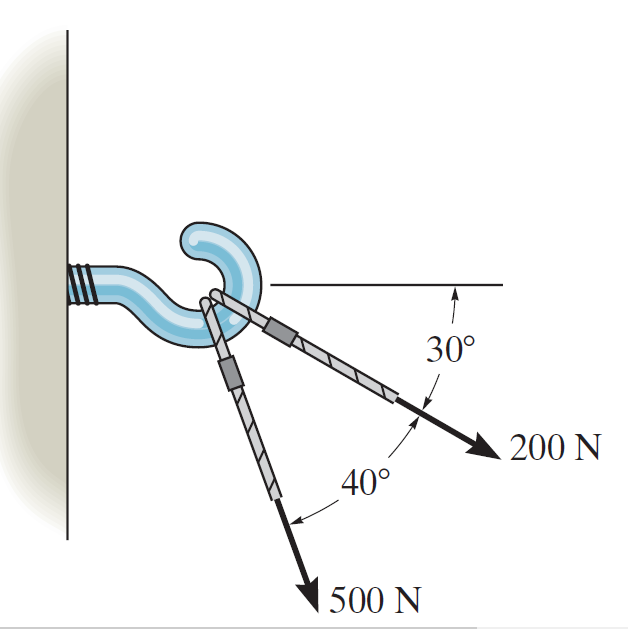$F_{R}=\sqrt{(200)^{2}+(500)^{2}-2(200)(50) \cos 140}$

$=666 N$

If $\theta=60^{\circ}$  and $T=5 \mathrm{kN}$, determine the magnitude of the resultant force acting on the eyebolt and its direction measured clockmise from the positive  $x$ axis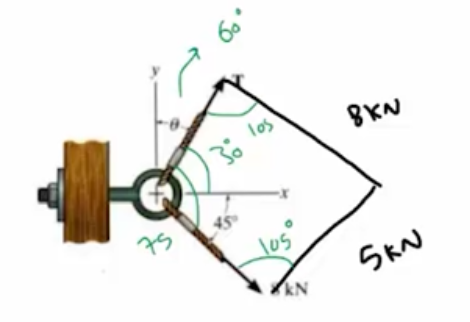$F_{R}=\sqrt{(5)^{2}+(8)^{2}-2(5)(8)\left(05 | 05^{\circ}\right.}$

$=10.47 \mathrm{kN}$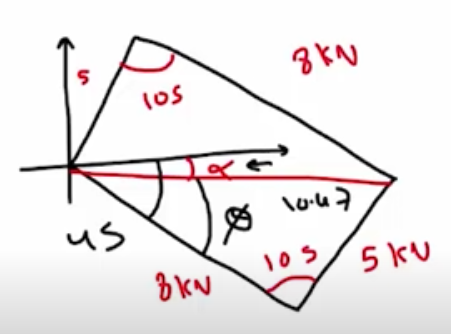$\frac{\sin \phi}{5}=\frac{\sin 105}{10.47}$

$\rightarrow \phi=27.5^{\circ}$

$∴ \alpha=45^{0}-27 \cdot 5=17.5^{\circ}$

Determine the angle of $\theta$ for connecting member $A$ to the plate so that the resultant force of $\mathrm{F}_{A}$ and $\mathrm{F}_{B}$ is directed horizontally to the right. Also, what is the magnitude of the resultant force?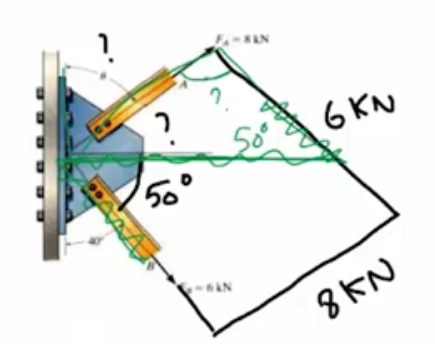$\frac{6}{\sin \phi}=\frac{8}{\sin 50}$

$∴ \phi=35.1^{\circ}$

$∴ \theta=90-35.1=54.9^{\circ}$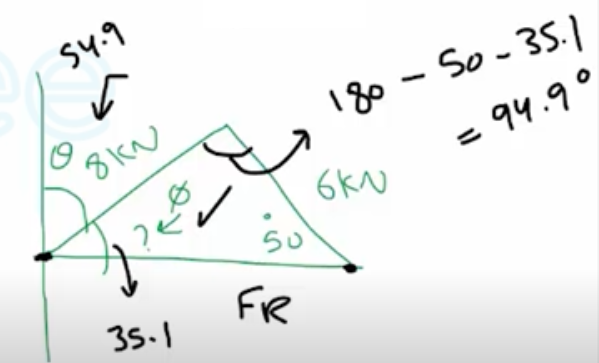$\frac{F_{R}}{\sin 94.9}=\frac{8}{\sin 50^{\circ}} \rightarrow F_{R}=10.4 k N$

No comments yet